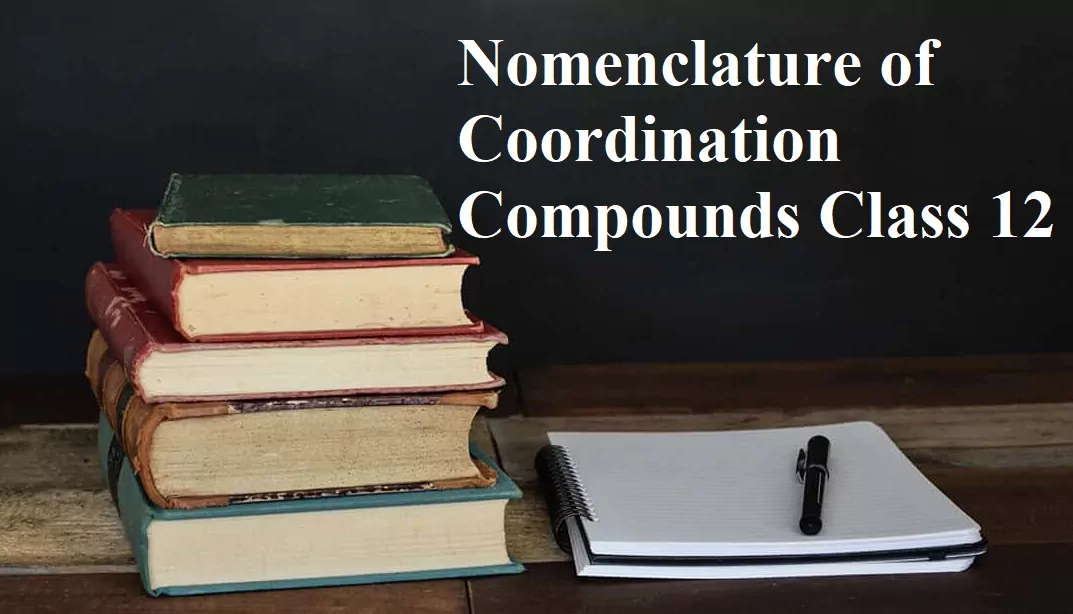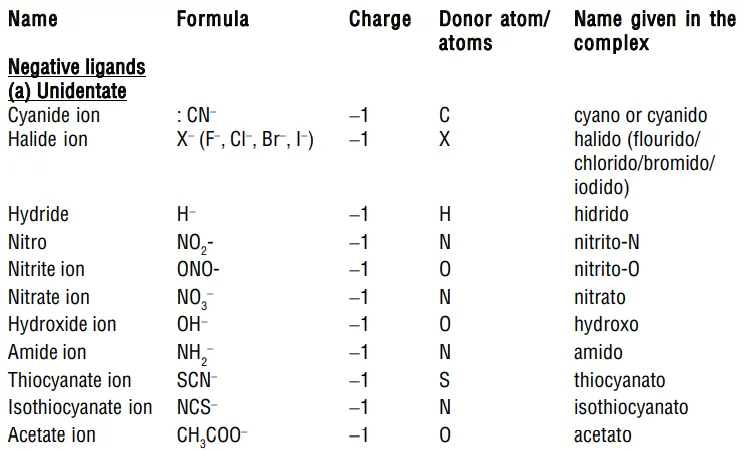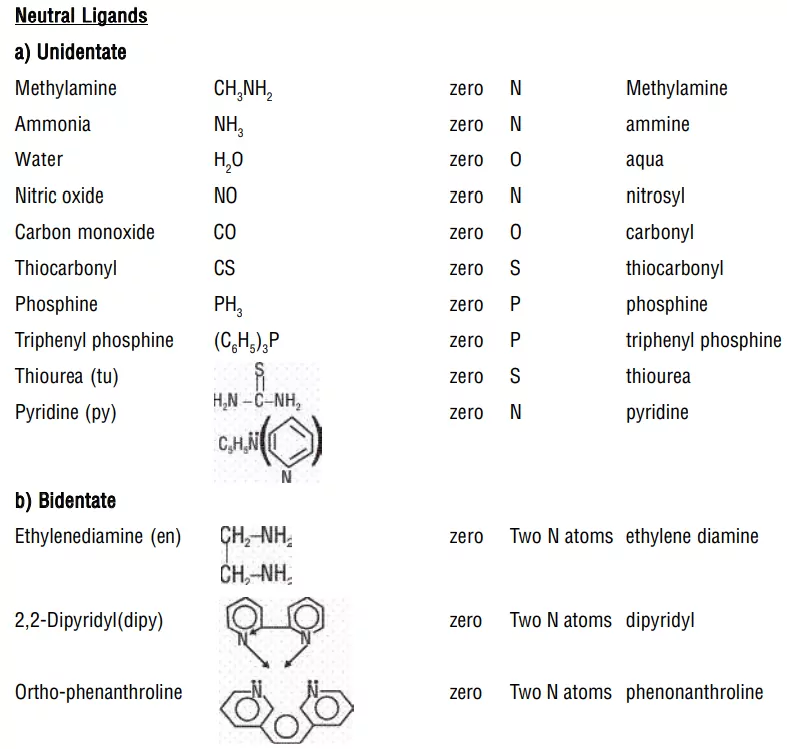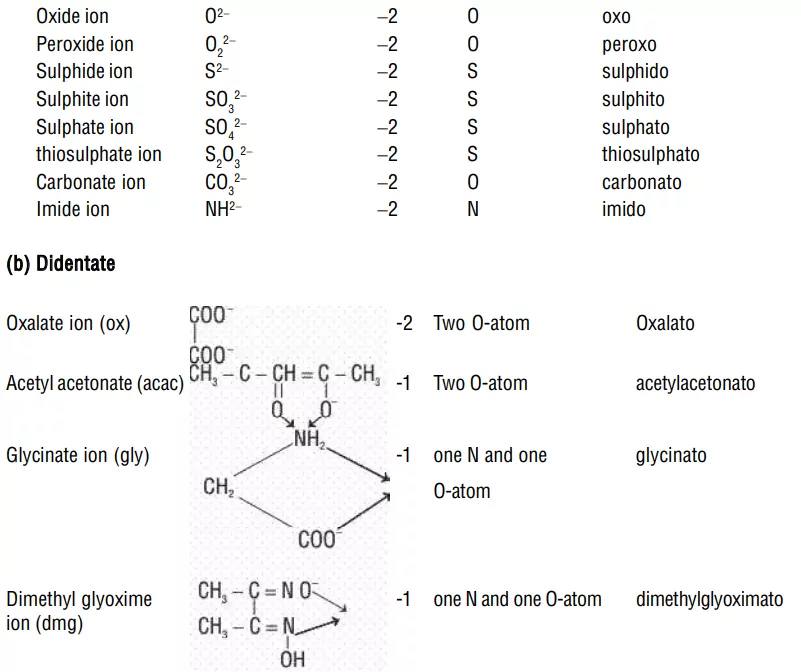# Nomenclature of Coordination Compounds Class 12Nomenclature of Coordination Compounds Class 12

Werner’s Theory of Coordination Compounds
Alfred Werner, a Swiss chemist proposed a theory about the nature of bonding in the coordination compounds (complexes) in the year 1893. The main postulates of this theory are:
1. Metals possess two types of linkages (valencies).
a) Primary or principal or ionizable links (valency) which are the same as the oxidation state of the metal.
b) Secondary or non-ionizable links (valency) which are the same as the coordination number of the central metal atom/ion. This number is fixed for a metal.
2. A metal atom satisfies both primary as well as secondary linkages (valencies). Primary linkages (valencies) are satisfied by negative ions whereas secondary linkages (valencies) are satisfied by ligands (neutral or negative ions/groups).
3. Ligands satisfying secondary linkages (valencies) are directed towards fixed directions (spatial arrangements) giving a definite geometry to the complex but primary valencies are non-directional. Such spatial arrangements are called coordination polyhedra.Nomenclature of Coordination Compounds Class 12

Rules for naming mononuclear coordination compounds:
1. Order of naming ions: The positive ion (cation) whether simple or complex, is named first followed by the negative ion (anion). The name is started with a small letter and the complex part is written as one word.
2. Naming ligands:
i) Negative ligands (organic or inorganic) end in −O, eg, CN (cyano), Cl (chlorido), Br (bromido), F (fluorido), NO2 (nitro), OH (hydroxo), O2− (oxo), H (hydrido). If the name of the anionic ligands ends in −ide, −ite or −ate, the last ‘e’ is replaced by ‘O’ giving −ido, −ito and −ato eg, SO42− (sulphato), C2O42− (oxalato), NH2 (amido), NH2 (imido), ONO (nitrito).
ii) Neutral ligands have no special ending, NH3 (ammine), H2O (aqua), CO (carbonyl)
iii) Positive ligands (which are very few) end in -ium, NO+ (nitrosoium), NO2+ (nitronium).
3. Numerical prefixes to indicate the number of ligands: If there are several ligands of the same type, the prefixes like di, tri, tetra, penta, and hexa are used to indicate the number of ligands of that type. When the name of polydentate ligand includes a number e.g., ethylenediamine, then bis, tris, tetrakis are used as prefixes.
4. Order of naming of ligands: All ligands whether negative, neutral or positive are named first in alphabetical order followed by the name of the metal atom/ion.
5. Naming of the complexion and ending of the central atom: Ligands are named first followed by the metal atom.
a) If the complexion is a cation or the coordination compound is non-ionic, the name of the central metal ion is written as such followed by its oxidation state indicated by a Roman numeral (such as II, III, IV) in the parentheses at the end of the name of the metal without any space between the two.
b) If the complexion is an anion, the name of the central metal atom is made to end in-ate followed by the oxidation number in brackets without any space between them.

Nomenclature of Coordination Compounds Class 12

Rules for Writing Formula from the Name of the Mononuclear Complex
(a) Formula of the cation (whether simple or complex) is written first, followed by the anion.
(b) The formula of the complexion (coordination entity) (whether charged or not) is written in square brackets called coordination sphere.
(c) Within the coordination sphere, the symbol of the metal atom is written first followed by the symbols/formulas of the ligands arranged alphabetically according to their names irrespective of the charge present on them. While listing the ligands alphabetically, the following rules should be followed:
i) Polydentate ligands are also listed in alphabetical order.
ii) The position of abbreviated ligands in the alphabetical order is determined from the first letter of the abbreviation.
iii) The position of ligands with special names (such as aqua for water) in alphabetical order is determined from the first letter of the special name.
iv) Abbreviations used for the ligands and the formulas of the polyatomic ligands are enclosed in parentheses separately.
v) The metal atom as well as all the ligands are listed without any space between them.
(d) If the formula of the complexion is to be written without writing the counter ion, the charge on the complexion is indicated outside the square bracket as a right superscript with the number before the sign. For example, [Fe(CN)6]3-, [Cu(NH3)4]2+, etc.
(e) The number of cations or anions to be written in the formula is calculated on the basis that the total positive charge must be equal to the total negative charge, as the complex as a whole is electrically neutral.
Example: Tetraammineaquachloridocobalt(III) chloride has complex ion [Co(NH3)4(H2O)Cl] and simple ion = Cl
To balance the charge, the formula will be [Co(NH3)4(H2O)Cl]Cl2.

Nomenclature of Coordination Compounds Class 12

Examples of Nomenclature of Coordination Compounds
1. [Cr(NH3)3(H2O)3]Cl3 – triamminetriaquachromium(III) chloride
Explanation: The complexion is inside the parentheses, which is a cation.
The ammine ligands are named before the aqua ligands according to alphabetical order.
Since there are three chlorides binding with the complexion, the charge on the complexion must be +3 (since the compound is electrically neutral).
From the charge on the complexion and the charge on the ligands, we can calculate the oxidation number of the metal. In this example, all the ligands are neutral molecules. Therefore, the oxidation number of chromium must be the same as the charge of the complexion, +3.

2. [Pt(NH3)5Cl]Br3 pentaamminechloroplatinum(IV) bromide
Explanation: The complexion is a cation, the counter anion is the 3 bromides.
The charge of the complexion must be +3 since it bonds with 3 bromides.
The NH3 are neutral molecules while the chloride carries -1 charge. Therefore, the oxidation number of platinum must be +4.

3. [Pt(H2NCH2CH2NH2)2Cl2]Cl2dichlorobis(ethylenediamine)platinum(IV) chloride
Explanation: ethylenediamine is a bidentate ligand, the bis- prefix is used instead of di-

4. [Co(H2NCH2CH2NH2)3]2(SO4)3 tris(ethylenediamine)cobalt(III) sulfate
Explanation: The sulfate is the counter anion in this molecule. Since it takes 3 sulfates to bond with two complex cations, the charge on each complex cation must be +3.
Since ethylenediamine is a neutral molecule, the oxidation number of cobalt in the complex ion must be +3.
Again, remember that you never have to indicate the number of cations and anions in the name of an ionic compound.

5. K4[Fe(CN)6] – potassium hexacyanoferrate(II)
Explanation: potassium is the cation and the complexion is the anion.
Since there are 4 K+ binding with a complexion, the charge on the complexion must be -4.
Since each ligand carries a –1 charge, the oxidation number of Fe must be +2.
The common name of this compound is potassium ferrocyanide.

6. Na2[NiCl4] – sodium tetrachloronickelate(II)
Explanation: The complexion is the anion so we have to add the suffix –ate in the name of the metal.

7. Pt(NH3)2Cl4 diamminetetrachloroplatinum(IV)
Explanations: This is a neutral molecule because the charge on Pt+4 equals the negative charges on the four chloro ligands.
If the compound is [Pt(NH3)2Cl2]Cl2, even then the number of ions and atoms in the molecule are identical to the example, it should be named: diamminedichloroplatinum(II) chloride, a big difference.

8. Fe(CO)5 pentacarbonyliron(0)
Explanations: Since it is a neutral complex, it is named in the same way as a complex cation. The common name of this compound, iron carbonyl, is used more often.

Explanation – The ligands here are Cl and H2O. Therefore, we will use the monodentate ligand names of “chloro” and “aqua”. Alphabetically, aqua comes before chloro, so this will be their order in the complex’s name. There are 4 aqua’s and 2 chloro’s, so we will add the number prefixes before the names. Since both are monodentate ligands, we will say “tetra[aqua]di[chloro]”.

10. (NH4)2[Ni(C2O4)2(H2O)2] – ammonium diaquabis(oxalato)nickelate(II)
Explanation: The oxalate ion is a bidentate ligand.

11. [Ag(NH3)2][Ag(CN)2] – diamminesilver(I) dicyanoargentate(I).

Nomenclature of Coordination Compounds Class 12

Some Other Examples:
1. [NiCl4]2−
2. Pt(NH3)2Cl4
3. [Pt(NH3)2Cl2]Cl2
4. [Co(NH3)5CO3]Cl
5. [Cr(H2O)4Cl2]Cl
6. [Zn(OH)4]−2
1. Tetrachloridonickelate(II) ion. The complexion, an anion, is inside the parentheses. We have to add the suffix -ate in the name of the metal.
2. Diamminetetrachloroplatinum(IV). This is a neutral molecule. The total charge on the ligands is -4. Therefore, the platinum oxidation number is +4.
3. Diamminedichloroplatinum(II) chloride. Here, the number of ions and atoms are the same. However, the brackets as well as the different oxidation number of the platinum result in a very different name.
4. Pentaamminecarbonatocobalt(III) chloride.
6. Tetra hydroxide zincate (II) ion.

Werner’s theory could not explain:
a) Only certain elements possess the remarkable property of forming coordination compounds?
b) The bonds in coordination compounds have directional characteristics.
c) Coordination compounds have characteristic magnetic and optical properties.

Nomenclature of Coordination Compounds Class 12